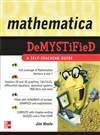# Mathematica Demystified: A Self-Teaching Guide

Need to learn MATHEMATICA? Problem SOLVED!

Take full advantage of all the powerful capabilities of Mathematica with help from this hands-on guide. Filled with examples and step-by-step explanations, Mathematica Demystified takes you from your very first calculation all the way to plotting complex fractals.

Using an intuitive format, this book explains the fundamentals of Mathematica up front. Learn how to define functions, create 2-D graphs of functions, write basic programs, and use modules. You'll move on to 3-D graphics, calculus, polynomial, linear, and differential equations, dynamical systems, and fractals. Hundreds of examples with concise explanations make it easy to understand the material, and end-of-chapter quizzes and a final exam help reinforce learning.

This self-teaching guide offers:

• A quick way to get up and running on Mathematica
• Coverage of Mathematica 6 and 7
• Tips for avoiding and correcting syntax errors
• Details on creating slideshow presentations of your work
• No unnecessary technical jargon
• A time-saving approach to performing better on an exam or at work!

Simple enough for a beginner, but challenging enough for an advanced user, Mathematica Demystified is your shortcut to mastering this fully integrated technical computing software.

# 下载地址

• 本书版权归原作者所有，本站暂无下载，请购买正版！
•